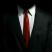﻿ CBC字节翻转攻击 - 七夕云黑帽博客-专注互联网络安全

### CBC字节翻转攻击

2020-11-14 22:43 作者：Mr.刘 | 网络安全 | 标签： CBC字节翻转攻击

## 实例分析

``````本组明文 = Decrypt(本组密文) ^ 上一组密文
A              B                 C
=========================================================
A = B ^ C
A ^ A = 0;   0 ^ A = A
C = A ^ A ^ C = B ^ C ^ A ^ C = A ^ B
(即C = A ^ B ，即：上一组密文 = 本组明文 ^ Decrypt(本组密文) )
ascii('a') ^ C ^ A ^ B = ascii('a') ^ A ^ B ^ A ^ B = ascii('a') ^ 0 = ascii('a')
(假设我们想要翻转成a，使用如上公式即可,即：想要的字符 = 上一组密文 ^ 本组明文 ^ Decrypt(本组密文) ^ 想要的字符 ) ``````

``````import urllib,base64,requests,re

r = requests.post(url,data=datas)
cipher = base64.b64decode(urllib.unquote(cipher))
offset = 9 new_cipher = cipher[:offset] + chr(ord(cipher[offset])^ord("A")^ord("a")) + cipher[offset+1:]

plain = base64.b64decode(re.findall("decode('(.*)')",r2.text))
old = plain[:len(iv)]
new = 'a:2:{s:8:"userna' new_iv = "".join([chr(ord(iv[i])^ord(old[i])^ord(new[i])) for i in xrange(16)])

print(r3.text) ``````

•文章作者：Mr.刘 作者QQ：905690245

﻿